# Math in Focus Grade 5 Chapter 7 Practice 5 Answer Key Comparing Three Quantities

Practice the problems of Math in Focus Grade 5 Workbook Answer Key Chapter 7 Practice 5 Comparing Three Quantities to score better marks in the exam.

## Math in Focus Grade 5 Chapter 7 Practice 5 Answer Key Comparing Three Quantities

Find the greatest common factor for each set of numbers.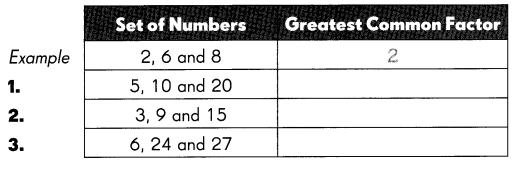Question 1.
5, 10 and 20
Answer: The greatest number that divides 5, 10 and 20 is 5, So Greatest common factor of 5,10 and 20 is 5.

Question 2.
3, 9 and 15
Answer: The greatest number that divides 3, 9 and 15 is 3, So Greatest common factor of 3, 9 and 15 is 3.

Question 3.
6, 24 and 27
Answer: The greatest number that divides 6, 24 and 27 is 3, So Greatest common factor of 6, 24 and 27 is 3.

Complete to express each ratio in simplest form.

Question 4.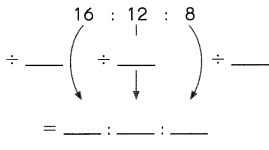Answer: 4 : 3 : 2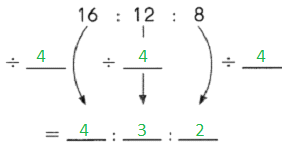Explanation:
Given,
All the numbers 16, 12 and 8 can be divided by 4 to get the simplest form in the ratio of 4 : 3 : 2.

Question 5.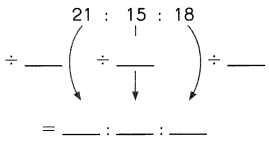Answer: 7 : 5 : 6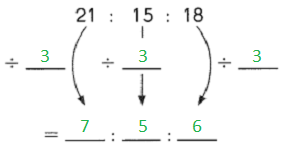Explanation:
Given,
All the numbers 21, 15 and 18 can be divided by 3 to get the simplest form in the ratio of 7 : 5 : 6.

Question 6.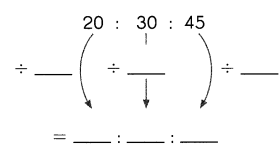Answer: 4 : 6 : 9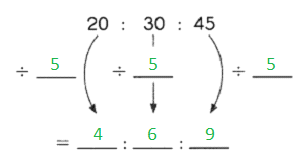Explanation:
Given,
All the numbers 20, 30 and 45 can be divided by 5 to get the simplest form in the ratio of 4 : 6 : 9.

Question 7.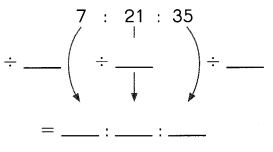Answer: 1 : 3 : 5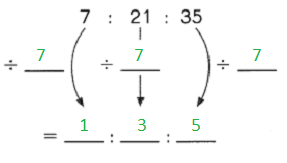Explanation:
Given,
All the numbers 7, 21 and 35 can be divided by 7 to get the simplest form in the ratio of 1 : 3 : 5.

Express each ratio in simplest form.

Question 8.
4 : 16 : 18 ___ : ___ : ___
All the numbers 4, 16 and 18 can be divided by 2 to get the simplest form in the ratio of 2 : 8 : 9.

Question 9.
27 : 12 : 21 __ : __ : ___
Given,
All the numbers 27, 12 and 21 can be divided by 3 to get the simplest form in the ratio of 9 : 4 : 7.

Question 10.
32 : 8 : 20 = ___ : ___ : ____
Answer: All the numbers 32, 8 and 20 can be divided by 4 to get the simplest form in the ratio of 8 : 2 : 5.

Question 11.
63 : 1 8 : 27 = ___ : ___ : ___
Answer: All the numbers 63, 18 and 27 can be divided by 9 to get the simplest form in the ratio of 7 : 2 : 3.

Complete.

Question 12.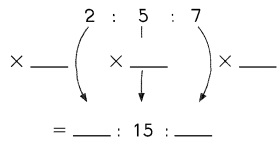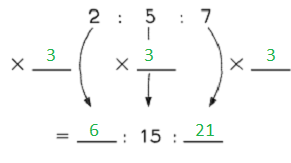All the numbers 2, 5 and 7 can be multiplied by 3 to get the simplest form in the ratio of 6 : 15 : 21.

Question 13.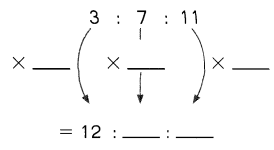Answer: All the numbers 3, 7 and 11 can be multiplied by 4 to get the simplest form in the ratio of 12 : 28 : 44.

Question 14.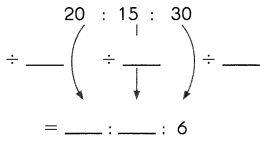Answer: All the numbers 20, 15 and 30 can be divided to get the simplest form in the ratio of 4 : 3 : 6.

Question 15.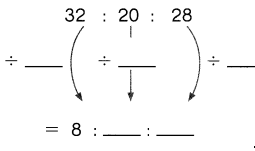Answer: All the numbers 32, 20 and 28 can be divided by 4 to get the simplest form in the ratio of 8 : 5 : 7.

Complete.

Question 16.
1 : 2 : 5 ___ : 6 : ___
Answer: All the numbers 1, 2 and 5 can be multiplied by 3 to get the ratio of 3 : 6 : 15.

Question 17.
7 : 4 : 3 = 28: ___ : ___
Answer: All the numbers 7, 4 and 3 can be multiplied by four to get the ratio of 28 : 16 : 12.

Question 18.
4 : 5 : 9 = ___ : 25 : ___
Answer: All the numbers 4, 5 and 9 can be multiplied by 5 to get the ratio of 20 : 25 : 45.

Question 19.
16 : 14 : 6 = ___ : ___ : 3
Answer: All the numbers 16, 14 and 6 can be divided by 2 to get the simplest form in the ratio of 8 : 7 : 3.

Question 20.
18 : 24 : 30 = ___ : 4 : ___
Answer: All the numbers 18, 24 and 30 can be divided by 6 to get the simplest form in the ratio of 3 : 4 : 5.

Question 21.
35 : 42 : 56 = 5 : ___ : ___
Answer: All the numbers 35, 42 and 56 can be divided by 7 to get the simplest form in the ratio of 5 : 6: 8.